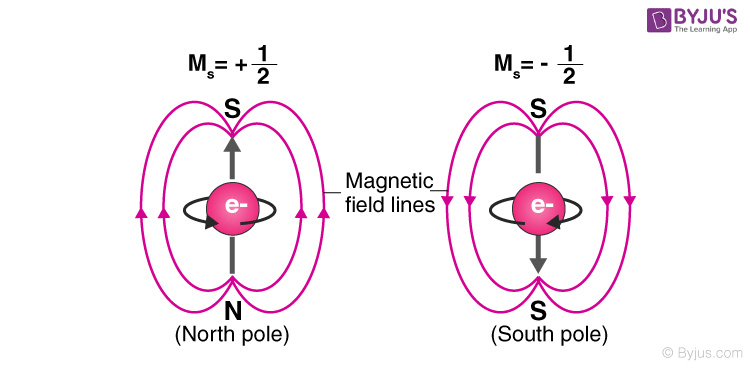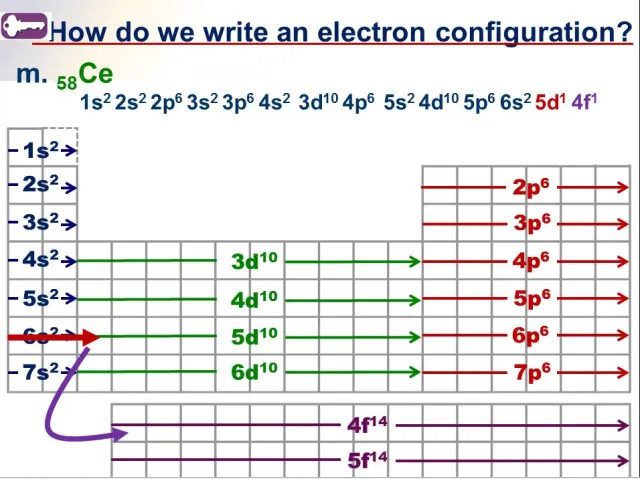Quantum numbers:

• Each electron has a set of four numbers, called quantum numbers

• Pauli Exclusion Principle

• Definition: no two electrons in the same atom can have the same set of four quantum numbers. Like an ID

• Principal quantum number (house) sublevel (floor of room) orbital (your room) electron spin (you in your room)Principle Quantum Number (n)

• Corresponds to energy level

• Describes the size of the electron cloud

• n = 1, 1st energy level, closest to nucleus

• n = 7, 7th energy level, farthest from nucleus

• As you move farther from the nucleus, energy increases.

• Energy levels are not evenly spaced out. The higher the energy level, the farther it’s spaced out

• To determine maximum number of electrons in an energy level, where n = energy level, 2n2

• Each energy level contains sublevels

Sublevel (l)

• Four sublevels that electrons can occupy:

n = 1

1 sublevel

s

n = 2

2 sublevels

s, p

n = 3

3 sublevels

s, p, d

n = 4

4 sublevels

s, p, d, f

• Properties:

Letter

Shape

# orientations

Max electrons

S

spherical

1

2

P

dumbell

3

6

D

double dumbbell (cloverleaf)

5

10

F

too complex

7

14

• Every orientation has a maximum of 2 electrons. (# orientations * 2 = max electrons)

Orbital (ml)

• Definition: the space where there is a high probability that it is occupied by a pair of electrons

• Describes the sublevels orientation in space

• Each orbital can hold 2 electrons

• Table:

Letter

# orbitals

# electrons

S

1

2

P

3

6

D

5

10

F

7

14

Electron Spin (ms)

• Describes the spin of an electron

• Tells exact locationProperties of Electrons:

• Electrons don’t behave like normal particles. They also act like waves. Because they act like waves, their exact location around the nucleus cannot be calculated.

• Bohr’s examination of the hydrogen atom also found that electrons were only allowed to have specific amounts of energy. Other energies are forbidden. The allowed energy of an electron around an atom is called a quantum.

• Table

Letter

Energy levels they can exist at

# orbitals

# electrons

S

1st and higher

1

2

P

2nd and higher

3

6

D

3rd and higher

5

10

F

4th and higher

7

14

Rules for filling orbitals:

• Aufbau Principle: Electrons always fill the lowest energy levels first.

• Electrons start at the bottom and work their way up

• Electrons fill orbitals the same way every time

• Pauli Exclusion Principle: No two electrons with the same energy characteristics can occupy an orbital at the same time

• One electron must be spinning up and the other electron must be spinning down

• Hund’s Rule: When filling multiple orbitals of the same sublevel (P, D, F) electrons half-fill the sublevel first before pairing electrons

Directions:• read the periodic table LEFT to RIGHT

• an S block has a maximum of 2 electrons

• a P block has a maximum of 6 electrons

• a D block has a maximum of 10 electrons

• an F block has a maximum of 14 electrons

• the exponent is the number of electrons

• stop once you get to the element

Ions:

• Cations - lose electrons

• become + ions

• usually metals

• to achieve the configuration of a noble gas

• Anions - gain electrons

• become - ions

• usually nonmetals

• to achieve the configuration of a noble gas

Electrons configuration:

metals go BACK to the LAST noble gas. non-metals go FORWARD to the NEXT noble gas

Lewis Dot Diagrams (non-hybridized)

• ONLY Valence electrons (s & p)

• Write the abbreviated configuration

• Fill in (s) first

• Draw in the (p) dots until you have a total of the # of valence electrons (left to right) OR you run out of (p)sOrbital Filling Diagrams

• Use a square, underscore, or circle. This represents an orbital

• Fill orbitals one at a time (like the Lewis Dot Diagram)